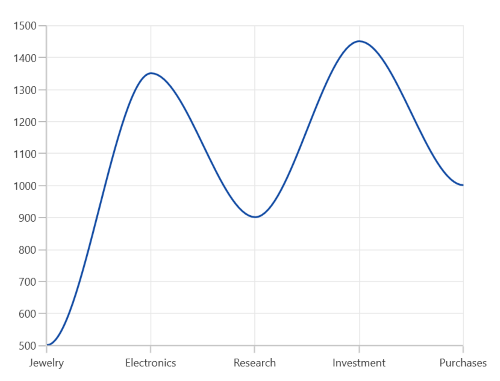# Line Chart in WinUI Chart (SfCartesianChart)

6 Dec 202211 minutes to read

## Line Chart

WinUI Line Chart is used to represent the data trends at equal intervals by connecting points on a plot with straight lines. To render a line chart, create an instance of LineSeries, and add it to the Series collection property of SfCartesianChart.

``````<chart:SfCartesianChart>

<chart:SfCartesianChart.XAxes>
<chart:CategoryAxis />
</chart:SfCartesianChart.XAxes>

<chart:SfCartesianChart.YAxes>
<chart:NumericalAxis />
</chart:SfCartesianChart.YAxes>

<chart:SfCartesianChart.Series>
<chart:LineSeries XBindingPath="Demand"
ItemsSource="{Binding Data}"
YBindingPath="Year2010"/>
<chart:LineSeries XBindingPath="Demand"
ItemsSource="{Binding Data}"
YBindingPath="Year2011"/>
</chart:SfCartesianChart.Series>

</chart:SfCartesianChart>``````
``````SfCartesianChart chart = new SfCartesianChart();
CategoryAxis xAxis = new CategoryAxis();
NumericalAxis yAxis = new NumericalAxis();

LineSeries series1 = new LineSeries()
{
ItemsSource = new ViewModel().Data,
XBindingPath = "Demand",
YBindingPath = "Year2010",
};

LineSeries series2 = new LineSeries()
{
ItemsSource = new ViewModel().Data,
XBindingPath = "Demand",
YBindingPath = "Year2011",

};

this.Content = chart;``````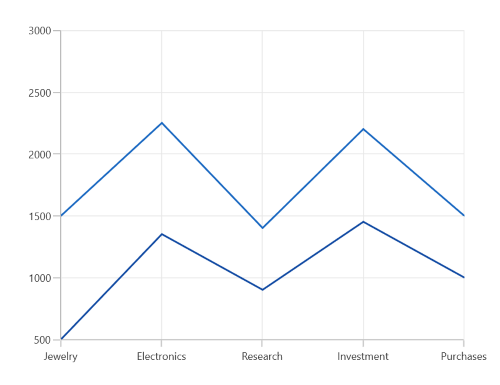### Dashed line

The StrokeDashArray property of LineSeries is used to render the line series with dashes. Odd value is considered as rendering size and even value is considered as gap.

``````<chart:SfCartesianChart>

<chart:SfCartesianChart.XAxes>
<chart:CategoryAxis />
</chart:SfCartesianChart.XAxes>

<chart:SfCartesianChart.YAxes>
<chart:NumericalAxis />
</chart:SfCartesianChart.YAxes>

<chart:SfCartesianChart.Series>
<chart:LineSeries XBindingPath="Demand"
ItemsSource="{Binding Data}"
YBindingPath="Year2010"
StrokeDashArray="5,2"/>
<chart:LineSeries XBindingPath="Demand"
ItemsSource="{Binding Data}"
YBindingPath="Year2011"
StrokeDashArray="5,2"/>
</chart:SfCartesianChart.Series>

</chart:SfCartesianChart>``````
``````SfCartesianChart chart = new SfCartesianChart();
CategoryAxis xAxis = new CategoryAxis();
NumericalAxis yAxis = new NumericalAxis();

DoubleCollection doubleCollection = new DoubleCollection();
. . .
LineSeries series1 = new LineSeries()
{
ItemsSource = new ViewModel().Data,
XBindingPath = "Demand",
YBindingPath = "Year2010",
StrokeDashArray = doubleCollection

};

LineSeries series2 = new LineSeries()
{
ItemsSource = new ViewModel().Data,
XBindingPath = "Demand",
YBindingPath = "Year2011",
StrokeDashArray = doubleCollection
};

this.Content = chart;``````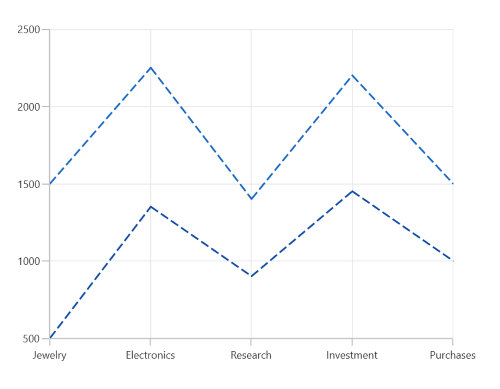## Step Line Chart

The StepLineSeries plots horizontal and vertical lines to connect the data points resulting in a step-like progression.

``````<chart:SfCartesianChart>

<chart:SfCartesianChart.XAxes>
<chart:CategoryAxis />
</chart:SfCartesianChart.XAxes>

<chart:SfCartesianChart.YAxes>
<chart:NumericalAxis />
</chart:SfCartesianChart.YAxes>

<chart:SfCartesianChart.Series>
<chart:StepLineSeries ItemsSource="{Binding Data}"
XBindingPath="XValue"
YBindingPath="YValue"/>
<chart:StepLineSeries ItemsSource="{Binding Data}"
XBindingPath="XValue"
YBindingPath="YValue1"/>
</chart:SfCartesianChart.Series>

</chart:SfCartesianChart>``````
``````SfCartesianChart chart = new SfCartesianChart();
CategoryAxis xAxis = new CategoryAxis();
NumericalAxis yAxis = new NumericalAxis();

StepLineSeries series1 = new StepLineSeries();
series1.ItemsSource = new ViewModel().Data;
series1.XBindingPath = "XValue";
series1.YBindingPath = "YValue";

StepLineSeries series2 = new StepLineSeries();
series2.ItemsSource = new ViewModel().Data;
series2.XBindingPath = "XValue";
series2.YBindingPath = "YValue1";

this.Content = chart;``````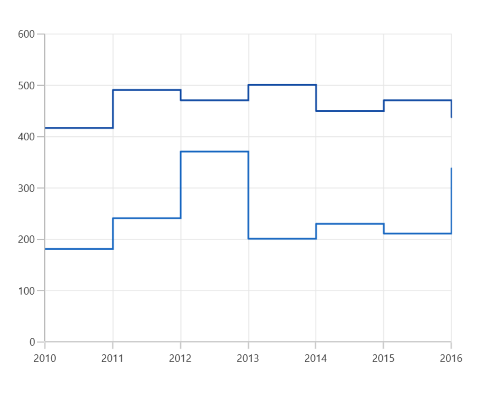NOTE

You can refer to our WinUI Step Line Chart feature tour page for its groundbreaking feature representations. You can also explore our WinUI Step Line Chart example that shows how to easily configure with built-in support for creating stunning visual effects.

## Spline Chart

The SplineSeries resembles line series, but instead of connecting the data points with line segments, the data points are connected by smooth bezier curves.

``````<chart:SfCartesianChart>

<chart:SfCartesianChart.XAxes>
<chart:CategoryAxis />
</chart:SfCartesianChart.XAxes>

<chart:SfCartesianChart.YAxes>
<chart:NumericalAxis />
</chart:SfCartesianChart.YAxes>

<chart:SfCartesianChart.Series>
<chart:SplineSeries XBindingPath="Demand"
ItemsSource="{Binding Data}"
YBindingPath="Year2010"/>
<chart:SplineSeries XBindingPath="Demand"
ItemsSource="{Binding Data}"
YBindingPath="Year2011"/>
</chart:SfCartesianChart.Series>

</chart:SfCartesianChart>``````
``````SfCartesianChart chart = new SfCartesianChart();
CategoryAxis xAxis = new CategoryAxis();
NumericalAxis yAxis = new NumericalAxis();

SplineSeries series1 = new SplineSeries()
{
ItemsSource = new ViewModel().Data,
XBindingPath = "Demand",
YBindingPath = "Year2010",
};

SplineSeries series2 = new SplineSeries()
{
ItemsSource = new ViewModel().Data,
XBindingPath = "Demand",
YBindingPath = "Year2011",
};

this.Content = chart;``````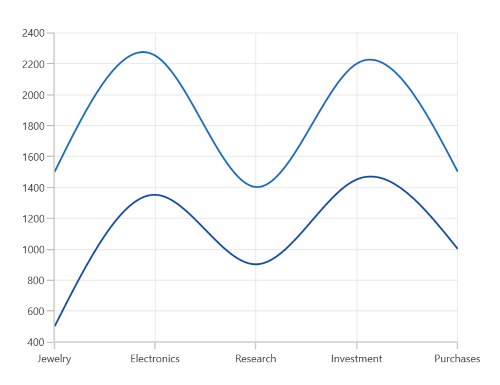### Spline rendering types

The Type property allows you to change the spline curve in series. By default, the value of the Type is Natural.

The following types are used in SplineSeries:

• `Natural`
• `Monotonic`
• `Cardinal`
• `Clamped`
``````<chart:SfCartesianChart>

<chart:SfCartesianChart.XAxes>
<chart:CategoryAxis />
</chart:SfCartesianChart.XAxes>

<chart:SfCartesianChart.YAxes>
<chart:NumericalAxis />
</chart:SfCartesianChart.YAxes>

<chart:SfCartesianChart.Series>
<chart:SplineSeries XBindingPath="Demand"
ItemsSource="{Binding Data}"
YBindingPath="Year2010"
Type="Cardinal"/>
</chart:SfCartesianChart.Series>

</chart:SfCartesianChart>``````
``````SfCartesianChart chart = new SfCartesianChart();
CategoryAxis xAxis = new CategoryAxis();
NumericalAxis yAxis = new NumericalAxis();

SplineSeries series = new SplineSeries()
{
ItemsSource = new ViewModel().Data,
XBindingPath = "Demand",
YBindingPath = "Year2010",
Type = SplineType.Cardinal
};